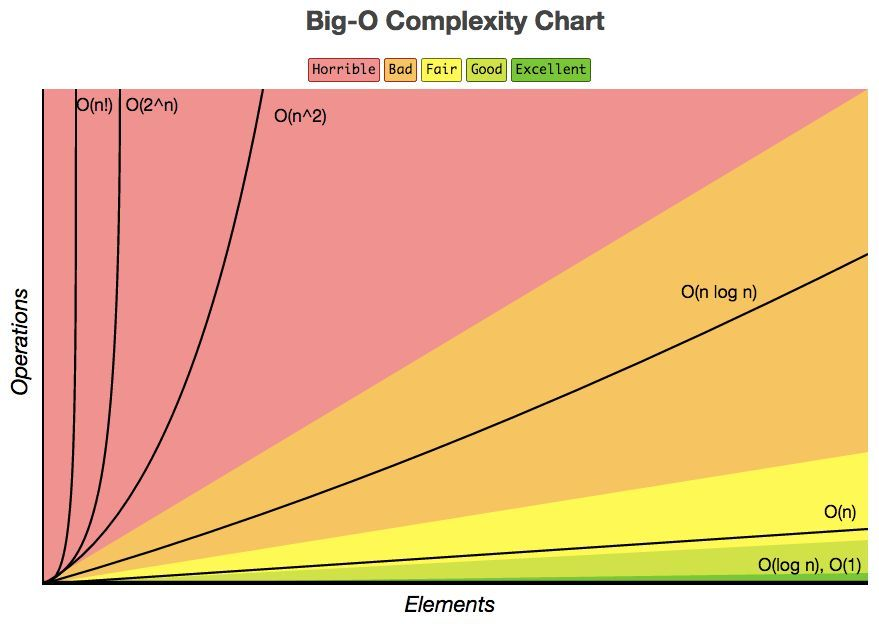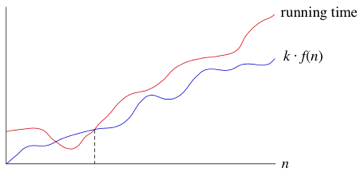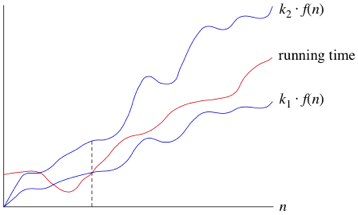# 不复杂的时间复杂度

2020-12-14 14:30:32 说人话## 大O表示法

int cal(int n) {
int sum = 0;
int i = 1;
for (; i <= n; i++) {
sum = sum + i;
}
return sum;
}


## 几种常见的时间复杂度量级

### 常数阶$$O(1)$$

int a = 1;
int b = 2;
int c = 3;


### 对数阶$$O(logn)$$、线性对数阶$$O(nlogn)$$

while (n > 1)
n = n/2


while (n > 1)
n = n/3


for (int i = 0; i < n; i++) {
while (n > 1)
n = n/2
}


### 平方阶$$O(n^2)$$、立方阶$$O(n^3)$$

int a = 0;
for (int i = 0; i < n; i++) {
for(int j = 0; j < n; j++) {
a++;
}
}


### 指数阶$$O(2^n)$$

for (int i = 0; i < 2^n; i++) {
n++;
}


### 阶乘阶$$O(n!)$$

int factorial(int n) {
for (int i = 0; i < n; i++) {
factorial(n - 1);
}
}


### 时间效率排名## 最好、最坏以及平均时间复杂度

// Tell whether the array a contains x.
boolean contains(int[] a, x) {
for (int i = 0; i < a.length; i++) {
if (x == a[i])
return true;
}
return false
}


$$T_1(n)$$, 设$$T_2(n)$$, ...分别为所有可能情况下的时间复杂度；设 $$P_1(n)$$, $$P_2(n)$$, ...为这些对应情况的分布概率。则平均时间复杂度（average-case time complexity）$$P_1(n)T_1(n) + P_2(n)T_2(n)+ ...$$

## $$\Omega$$ 表示法

$$\Omega$$表示法$$\Omega(f(n))$$表示的是渐进下界（lower bound），即当数据规模越来越大时，算法的执行时间最少也不会小于$$k \cdot f(n)$$（k为某一常数）。## $$\Theta$$表示法

$$\Theta$$表示法$$\Theta(f(n))$$表示的是渐进紧确界（tight bound），即当数据规模越来越大时，算法的执行时间将落在$$k_1 \cdot f(n)$$$$k_2 \cdot f(n)$$之间（$$k_1,k_2$$均为常数）。## Q & A

• A盒子有10到20只小虫子。
• B盒子有30到40只小虫子。
• 两个盒子在外观上一模一样，所以小明分不清哪个是A盒子，哪个是B盒子。
• 小明必须选出一个盒子，并吃掉里面所有的虫子。

## 参考

https://www.cnblogs.com/linj7/p/14133072.html